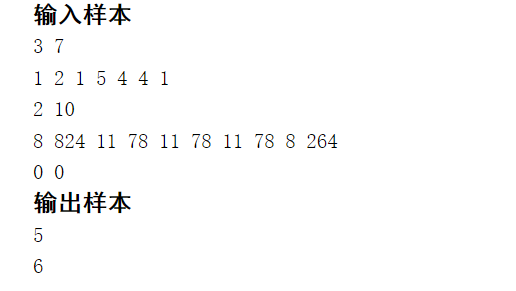# （python）小最的电脑上安装了一个机器翻译软件,他经常用这个软件来翻译英语文章这个翻译软件的原理很简单,它只是从头到尾,依次将每个英文单词用对应的中文含义来替换。对于每个英文单词,软件会先在内存中M=int(input('M='))#内存
N=int(input('N='))#文章长度
listN=[]
i=0
while i<N:
N1 = input('输入文章：')#输入文章一次只需要输入一个
listN.append(N1)
i+=1
listm=[]
sum=0
for i in listN:
if i in listm:
pass
else:
if len(listm)>M:
del listm
sum=sum+1
listm.append(i)
else:
sum=sum+1
listm.append(i)
c=' '.join(listN)#转化格式，用空格链接
print(c)
print(sum)

11-26
11-03107603-24148
05-09
12-03391
09-16521
06-03243
01-31103
04-272401